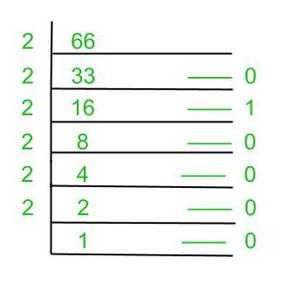Open in App
Not now

# How to convert Decimal to Hexadecimal?

• Last Updated : 31 Mar, 2022

The number system is the system of representing numbers. There are different types of representations in the number system. They are Binary (Base – 2), Decimal (Base – 10), Octal (Base – 8), and Hexadecimal (Base – 16).

Decimal to hexadecimal conversion is the process of converting a decimal number to a hexadecimal number. The decimal number has a base value of 10 (0 to 9) and the hexadecimal has a base value of 16 (0 to 9 and A to F for 10-15).

The following table shows the representation of Hexadecimal, decimal and binary values:

There are different ways to convert Decimal to Hexadecimal numbers. They are as follows:

### Converting Numbers with the Integer part

Step 1: Take the decimal number as dividend and 16 as the divisor (hexadecimal number will have 16 as a base)

Step 2: Divide the dividend with the divisor and store the remainder in an array

Step 3: Now divide the quotient obtained from the above step by 16 and store the remainder in the array.

Step 4: Repeat the third step until the number is greater than zero.

Step 5: The final hexadecimal value will be the reverse order of the array.

Example 1: Let’s consider a decimal number 450. We need to convert this decimal number to a hexadecimal number.

Solution:

Given: Decimal number = 450(10)

Step 1: 450/16 gives Q1 = 28 and R1 = 2

Step 2: 28/16 gives Q2 = 1 and R2 = 12 = C

Step 3: 1/16 gives Q3 =  0 and R3 = 1

Step 4: 0/16 gives Q4 =  0 and R4 = 0

Therefore, the hexadecimal value is 01C2(16)

Example 2: Convert 6096(10) to ________(16)

Solution:

Given: Decimal number = 6096(10)

Step 1: 6096/16 gives Q1 = 381 and R1 = 0

Step 2: 381/16 gives Q2 = 23 and R2 = 13 = D

Step 3: 23/16 gives Q3 =  1 and R3 = 7

Step 4: 1/16 gives Q4 =  0 and R4 = 1

Step 5: 0/16 gives Q5 =  0 and R5 = 0

Therefore, the hexadecimal value is 017D0(16) or 17D0(16)

### Converting Numbers with Fractional parts

Step 1: Take the decimal fractional number and multiply it with 16 (hexadecimal number will have 16 as a base)

Step 2: Store the remainder in an array i.e. the integer part

Step 3: Repeat the above two steps until the number is zero.

Step 4: The final hexadecimal value will be the elements of the array.

Example 1: Convert 0.0568(10) to _______(16)

Solution:

Given: Decimal number = 6096(10)

Step 1: 0.0645 x 16 = 1.032 and R1 = 1

Step 2: 0.032 x 16 = 0.512 and R2 = 0

Step 3: 0.512 x 16 = 8.192 and R3 = 8

Step 4: 0.192 x 16 = 3.072 and R3 = 3

Step 5: 0.072 x 16 = 1.152 and R3 = 1

The fractional part is still not zero so it continues, now we can take up to 5 remainders

Therefore, the hexadecimal value is 0.10831…(16)

### Converting Numbers with Both Integer and Fractional parts

Steps of both the integer part and fractional part are to be followed.

Example 1: Convert 256.00390625(10) to _________(16)

Solution:

Given: Decimal number = 256.00390625(10)

Let’s perform the conversion on integer part:

Integer value = 256(10)

Step 1: 256/16 gives Q1 = 16 and R1 = 0

Step 2: 16/16 gives Q2 = 1 and R2 = 0

Step 3: 1/16 gives Q3 =  0 and R3 = 1

Let’s perform the conversion on fractional part:

Fractional value = 0.00390625(10)

Step 1: 0.00390625 x 16 = 0.0625 and R1 = 0

Step 2: 0.0625 x 16 = 1.0 and R2 = 1

Step 3: 0.0 x 16 = 0 and R3 = 0

Therefore, the hexadecimal value is 100.010(16)

### Indirect Conversion

In this type of conversion, we will convert the decimal number to a binary number or octal number and further convert it to a hexadecimal number by grouping digits.

Example 1: Convert 66(10) to _______(16)

Solution:

Given: Decimal Number =  345(10)

Convert the given decimal number to its binary form:Binary Number = 1000010(2)

Now, Group 4 binary digits as one group and write its hexadecimal value

i.e. 0100 0010